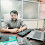# Complete Program Explanation of Java

4

class Hello
{
public static void main(String abc[])
{
System.out.println("hello world");
}
}
//class is the blueprint of the object, it is used to define the functionality of the object using data member and member function. we can define a single object and multiple objects definition both using class.
{}   --->  it is called token of class which is used to define block, all block-level statements will be defined by this. for example if-else, switch, loop, function, etc
public:-   it is an access specifier or access modifier that is used to define the scope of the data member, member function, and class, the public can be accessible outside of the project, package, method, and class.
static:- it is called a non-access modifier of java that provides compile-time memory allocation means if we create a static variable then its memory will be fixed but a value can be changed, static variable, and method will be called without any object.
void:-  it is a data type that returns the null value in java.
main():-   it is a predefined entry-point function that will be mandatory for program execution.main() always will be declared static because it will be called without any object. implicit.
String args[]:- args is the argument that is used to contain String array type data using the command line, if we want to pass data from command line input then it will work. String type array is mandatory in the main() as a parameter.
args is the user define identifier, it names can be changed.
System:-  It is the predefined class of java that exists under java.lang package .it is used to handle input /output operations using Java.
out:-   It is the reference of PrintStream class which is used to print statements using print() or println().
print() or println():-  It is used to print output data of java. It is the method of the PrintStream class.
"hello world":-  It is String type literal
;   it means the end of the statement
Tags

1.solution of area of tringle using heron's formula.
public class Main
{
public static void main(String[] args) {
int a=3,b=4,c=5;
int s;
s=(a+b+c)/2;
int r;
r=s*(s-a)*(s-b)*(s-c);
System.out.println(Math.sqrt(r));
}
}

2.Command Line

class Terminal
{
public static void main(String args[])
{
System.out.println(args + " "+ args);

}

}

3.//Yogesh seroke
public class Main
{
public static void main(String[] args) {
int a=3,b=4,c=5;
int s;
s=(a+b+c)/2;
int r;
r=s*(s-a)*(s-b)*(s-c);
System.out.println(Math.sqrt(r));
}
}

4.//Prachi Malvi
class Areaoftriangle
{
public static void main(String args[])
{
double a,b,c,s,Area;
a = 5;
b = 6;
c = 2;
s = (a+b+c)/2;
Area = Math.sqrt(s*(s-a)*(s-b)*(s-c));
System.out.println(Area);
}
}